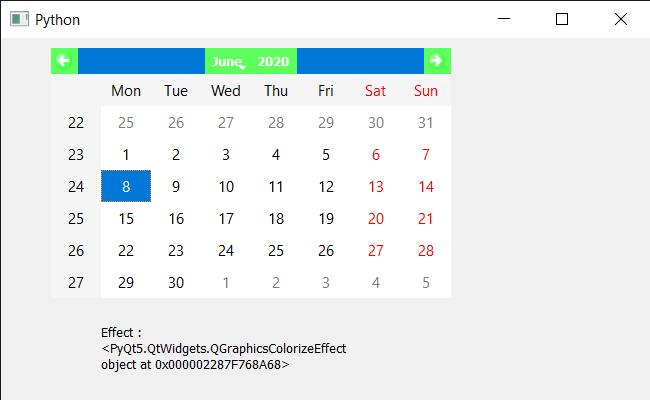Related Articles

# PyQt5 QCalendarWidget – Getting Graphics effect

• Last Updated : 09 Jun, 2020

In this article we will see how we can get the graphic effect of the QCalendarWidget. A graphic effect is an effect that can be used on a sprite or the Stage, changing their look in some way. Graphic effect can be color shadow anything. It can be set to calendar with the help of `setGraphicsEffect` method.

In order to do this we will use `graphicsEffect` method with the QCalendarWidget object.

Syntax : calendar.graphicsEffect()

Argument : It takes no argument

Return : It return QGraphicEffect object

Below is the implementation

 `# importing libraries``from` `PyQt5.QtWidgets ``import` `*` `from` `PyQt5 ``import` `QtCore, QtGui``from` `PyQt5.QtGui ``import` `*` `from` `PyQt5.QtCore ``import` `*` `import` `sys`` ` ` ` `class` `Window(QMainWindow):`` ` `    ``def` `__init__(``self``):``        ``super``().__init__()`` ` `        ``# setting title``        ``self``.setWindowTitle(``"Python "``)`` ` `        ``# setting geometry``        ``self``.setGeometry(``100``, ``100``, ``650``, ``400``)`` ` `        ``# calling method``        ``self``.UiComponents()`` ` `        ``# showing all the widgets``        ``self``.show()`` ` `    ``# method for components``    ``def` `UiComponents(``self``):`` ` `        ``# creating a QCalendarWidget object``        ``self``.calendar ``=` `QCalendarWidget(``self``)`` ` `        ``# setting geometry to the calender``        ``self``.calendar.setGeometry(``50``, ``10``, ``400``, ``250``)`` ` `        ``# setting cursor``        ``self``.calendar.setCursor(Qt.PointingHandCursor)`` ` `        ``# creating a color graphic effect``        ``color ``=` `QGraphicsColorizeEffect()``        ``color.setColor(Qt.green)`` ` `        ``# setting graphics to the calendar``        ``self``.calendar.setGraphicsEffect(color)`` ` `        ``# creating label to show the properties``        ``self``.label ``=` `QLabel(``self``)`` ` `        ``# setting geometry to the label``        ``self``.label.setGeometry(``100``, ``280``, ``250``, ``60``)`` ` `        ``# making label multi line``        ``self``.label.setWordWrap(``True``)`` ` `        ``# getting graphics effect``        ``value ``=` `self``.calendar.graphicsEffect()`` ` `        ``# setting text to the label``        ``self``.label.setText(``"Effect : "` `+` `str``(value))`` ` ` ` ` ` `# create pyqt5 app``App ``=` `QApplication(sys.argv)`` ` `# create the instance of our Window``window ``=` `Window()`` ` `# start the app``sys.exit(App.``exec``())`

Output :Attention geek! Strengthen your foundations with the Python Programming Foundation Course and learn the basics.

To begin with, your interview preparations Enhance your Data Structures concepts with the Python DS Course. And to begin with your Machine Learning Journey, join the Machine Learning – Basic Level Course

My Personal Notes arrow_drop_up